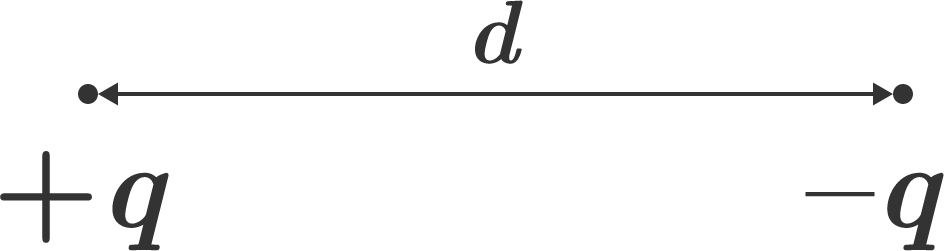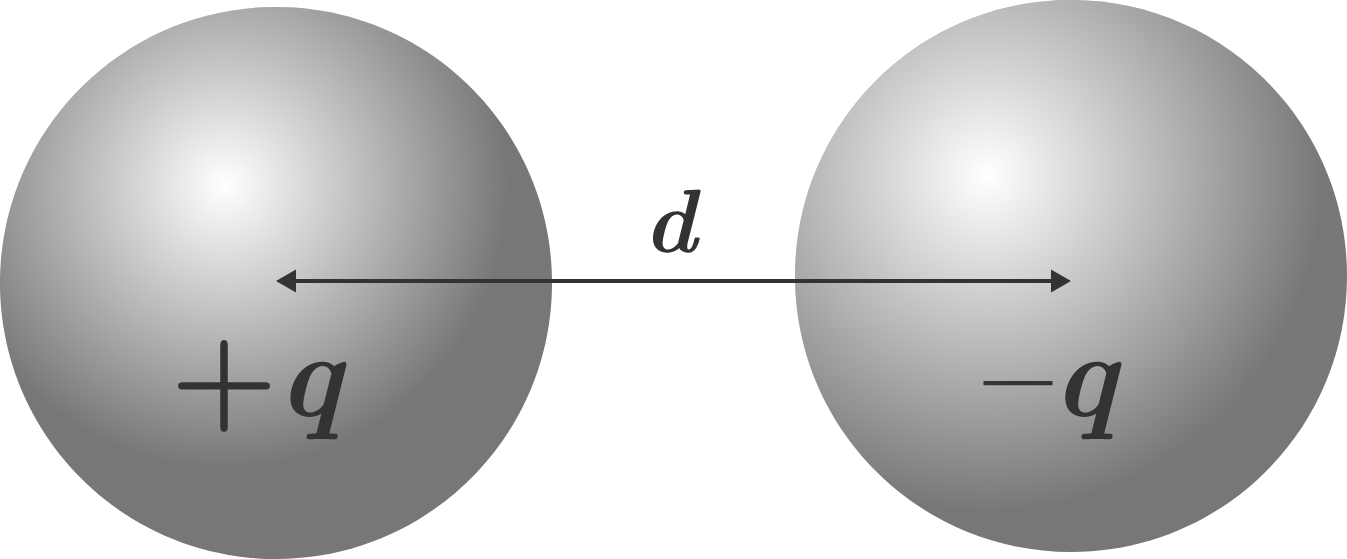# It’s scandalous!If two point charges $+q$ and $-q$ are separated by distance $d,$ then according to Coulomb's law, the attractive force between them is

$F_e=k_e\frac{q^2}{d^2},$

where $k_e$ is Coulomb's constant. But what if the charges were distributed on spheres instead of at points? Two charged conducting spheres with charges $+q$ and $-q$ are separated by center-to-center distance $d.$The attractive force between them is $\text{\_\_\_\_\_\_\_\_\_\_}.$

×